# Fraction

This format displays decimal numbers as actual fractions.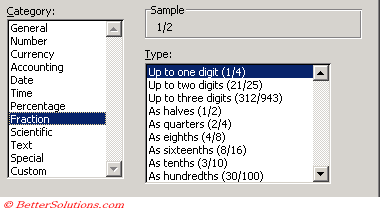All numbers are rounded to the nearest single digit fraction.

### Up to one digit (1/4)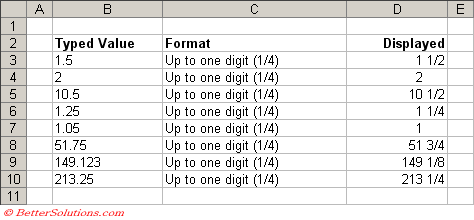### Up to two digits (21/25)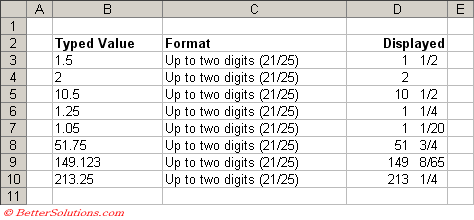### Up to three digits (312/943)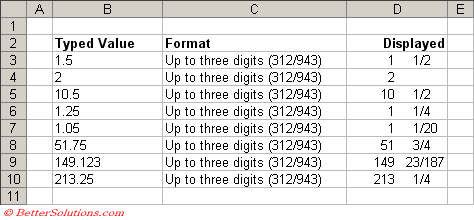### As halves (1/2)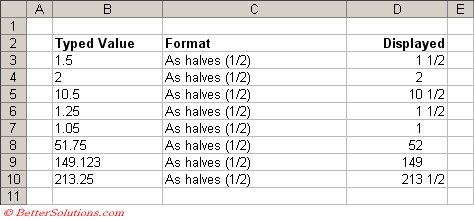### As quarters (2/4)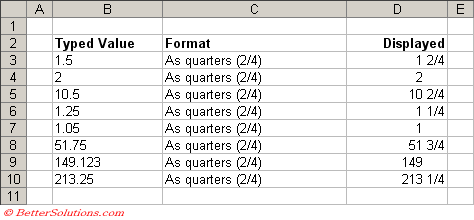### As eighths (4/8)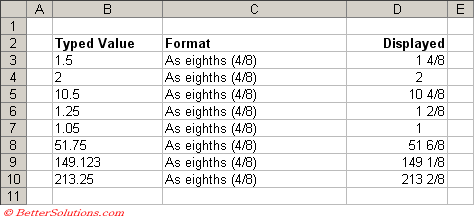### As sixteenths (8/16)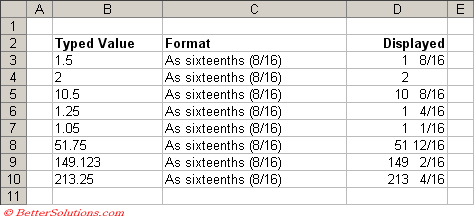### As tenths (3/10)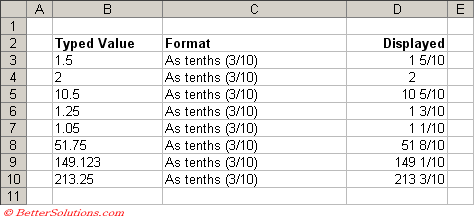### As hundredths (30/100)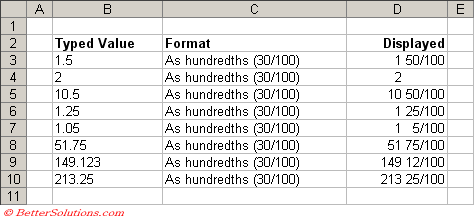### Entering Integer Fractions

To enter a fraction, type the whole number followed by a space and then the fraction.
You can use the slash character " / " when you enter your fraction.
Excel will automatically format the number as fraction.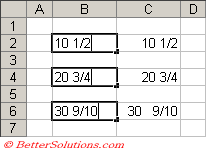This displays your number as a fraction instead of a number with decimal places.
Excel will convert any numbers you enter and display them as a fraction
You don't have to enter the number as a fraction but you can (using the backslash) i.e. you could enter 1/2

To display 1/2 you can either type 0.5 or 1/2

This format is often used for stock market quotes and can also be useful with certain types of measurements.
Excel will always try to calculate the closest fraction which depends on a few things:
1) whether the fraction actually exists
2) the type of rpecision level you have chosen
SS
3 digits
2 digits
1 digit

### Entering Non Integer Fractions

If you want to enter just a fraction with no whole number then just enter a zero followed by a space before entering the fraction.
If you forget to enter a zero when you are entering just a fraction with no whole number it will be formatted as text.
If you forget to enter a space after the zero the number will be formatted as a date.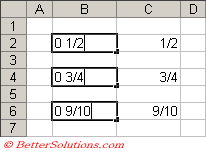### Displaying Fractions

If you have a decimal number and would like to display it as a fraction, select (Format > Cells)(Number tab) and select the Fraction category.
There are 9 built-in fraction number formats that you can use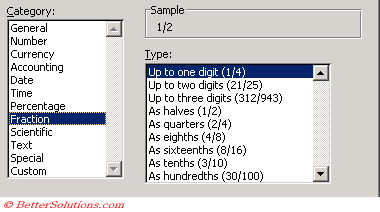You can also use a custom number format to create other Fraction formats.
The custom number format # ??/50 displays a value in 50ths.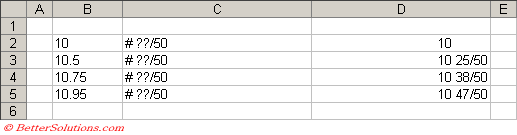The custom number format # ??/16\" displays a value in 16ths with a quotation mark appended at the end.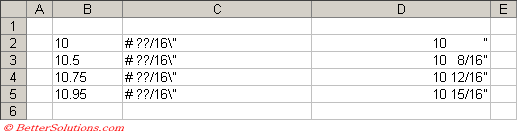The custom number format 0 "pounds and "??/100 "pence" combines text and a value expressed in fractional pounds.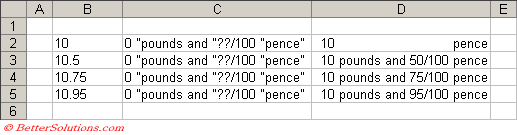### Important

Excel can sometimes interpret your fraction as a date or as text so always check the value afterwards.
To make sure that Excel does not interpret faction as a date, precede the fraction with a zero and a space.
All numbers are rounded to the nearest single digit fraction.